# LAKHMIR SINGH AND MANJIT KAUR Solutions for Class 10 Physics Chapter 2 - Magnetic Effects of Electric Current

Page / Exercise

## Chapter 2 - Magnetic Effects of Electric Current Exercise 73

Solution 3
Magnetic field is the strongest at the place where the magnetic field lines are closest together.
Solution 5

A compass needle gets deflected when brought near a bar magnet because the bar magnet exerts a magnetic force on the compass needle, which is itself a tiny pivoted magnet.

Solution 11

Because the strength of magnetic field produced by the cable is quite weak.

Solution 12
(a) The magnetic field lines are the lines drawn in a magnetic field along which a north magnetic pole would move. The magnetic field lines are also known as magnetic lines of forces.

Activity to draw a magnetic field line outside a bar magnet from one pole to another pole:
• Take a small compass and a bar magnet.
• Place the magnet on a sheet of white paper fixed on a drawing board, using some adhesive material.
• Mark the boundary of the magnet.
• Place the compass near the north pole of the magnet. The south pole of the needle points towards the north pole of the magnet. The north pole of the compass is directed away from the north pole of the magnet.
• Mark the position of two ends of the needle.
• Now move the needle to a new position such that its south pole occupies the position previously occupied by its north pole.
• In this way, proceed step by step till you reach the south pole of the magnet .
• Join the points marked on the paper by a smooth curve. This curve represents a magnetic field line.

(b) A freely suspended magnet points in the north-south direction because earth behaves as a magnet with its south pole in the geographical north and the north pole in the geographical south.

Solution 1
Prop of magnetic field lines:

(i) The magnetic field lines originate from the north pole of a magnet and end at its south pole.

(ii) The strength of magnetic field is indicated by the degree of closeness of the field lines. Where the field lines are closest together, the magnetic field is the strongest there.
Solution 2
(i) By using iron filings

(ii) By using compass
Solution 4

False

The axis of earth's imaginary magnet is inclined at an angle of 15o with the geographical axis.

Solution 6

Manufacturers use a magnetic strip in the refrigerator's door to keep it closed properly.

Solution 7
(a) north; south

(b) bar; south
Solution 8Magnetic field lines around a bar magnet
Solution 9

The space surrounding a magnet in which magnetic force is exerted, is called a magnetic field. The direction of magnetic field lines at a place can  be determined by using a compass needle. A compass needle placed near a magnet gets deflected due to the magnetic force exerted by the magnet. The north end of the needle of the compass indicates the direction of magnetic field at the point where it is placed.

Solution 10

Two magnetic field lines do not intersect each other due to the fact that the resultant force on a north pole at any point can be only in one direction. But if the two magnetic lines get intersect one another, this means that resultant force on a north pole placed at the point of interection will be along two directions, which is not possible.

## Chapter 2 - Magnetic Effects of Electric Current Exercise 74

Solution 24As the north pole of the magnetic needle is pointing in the opposite direction,so the nearer end of the magnet will be north pole.
Solution 25
(a)(b) X, as it repels the north pole (tip) of magnetic needle.
Solution 26

A=N; B=N; C=S; D=S; E=N; F=S

Solution 27

(i)(ii) Magnet 2 is weaker.

## Chapter 2 - Magnetic Effects of Electric Current Exercise 81

Solution 1

Magnetic effect

Solution 2

We conclude that a current carrying wire produces a magnetic field around it.

## Chapter 2 - Magnetic Effects of Electric Current Exercise 82

Solution 3

Magnetic effect of current was discovered by Oersted.

Solution 4

Magnetic field becomes very strong.

Solution 6
The magnetic field lines around a straight current-carrying conductor are concentric circles whose centres lie on the conductor.
Solution 7

Maxwell's corkscrew rule.

Solution 8

True

Solution 9

Solenoid

Solution 10
The strength of an electromagnet can be increased by

(i) increasing the number of turns in the coil

(ii) increasing the current flowing in the coil

(iii) reducing the length of air gap between the poles
Solution 11

(a) magnetic field; concentric circles

(b) bar magnet

(c) turns; current; iron

(d) north

(e) south

Solution 12

A current-carrying wire concealed in a wall can be located due to the magnetic effect of current by using a plotting compass. If a plotting compass is moved on a wall, its needle will show deflection at the place where current-carrying wire is concealed.

Solution 13We take a thick insulated copper wire and fix it in such a way that the portion AB of the wire is in the north-south direction as shown in fig. A plotting compass M is placed under the wire AB. The two ends of the wire are connected to a battery through a switch. When no current is flowing in the wire AB, the compass needle is parallel to the wire AB and points in the usual north-south direction. When current is passed through wire AB by closing the switch, we find that the compass needle is deflected from its north-south position. On opening the switch, the compass needle returns to its original position.

Thus, the deflection of compass needle by the current carrying wire shows that magnetic field is associated with an electric current.

Solution 14

(a)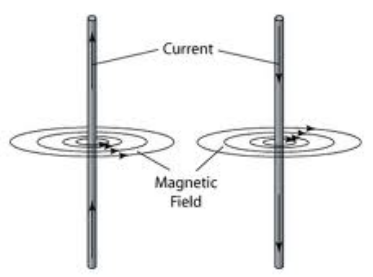(b) Maxwells right-hand thumb rule: According to this rule, imagine that you are grasping the current-carrying wire in your right hand so that your thumb points in the direction of current, then the direction in which your fingers encircle the wire will give the direction of magnetic field lines around the wire.

Solution 15

(b) According to Maxwell's right hand thumb rule: Imagine that you are grasping the current-carrying wire in your right hand so that your thumb points in the direction of current, then the direction in which your fingers encircle the wire will give the direction of magnetic field lines around the wire.Let AB be the straight wire carrying current in the vertically upward direction from A to B. To find out the direction of the magnetic field lines produced by this current, we imagine that we are grasping the current carrying wire in our right hand as shown in fig. so that our thumb points in the direction of current towards B. Now, the direction in which our fingers are folded gives the direction of magnetic field lines. In this case, the direction of magnetic field lines is in the anticlockwise direction.

Solution 16

According to Maxwell's corkscrew rule: Imagine driving a corkscrew in the direction of current, then the direction in which we turn its handle is the direction of magnetic field.

This rule is used to determine the direction of magnetic field around a straight current carrying conductor.

Solution 17
(a)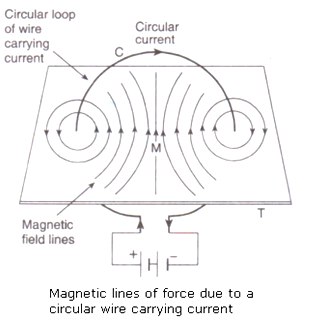(b) The strength of magnetic field produced by a current-carrying circular coil can be increased by:

-increasing the number of turns of wire in the coil

-increasing the current flowing through the coil
Solution 18

According to the Clock face rule, we look at one face of a circular wire (or coil) through which a current is passing:

(i) If the current around the face of the circular wire (or coil) flows in the clockwise direction, then that face of the circular wire will be South pole (S-pole).

(ii) If the current around the face of the circular wire (or coil) flows in the anti-clockwise direction, then that face of the circular wire will be North pole (N-pole).Solution 19

The strength of magnetic field produced by a current-carrying solenoid depends on:

1. The strength of current in the solenoid: Larger the current passed through solenoid, stronger will be the magnetic field produced.

2. The number of turns in the solenoid: Larger the number of turns in the solenoid, greater will be the magnetic field produced.

Solution 20
(a)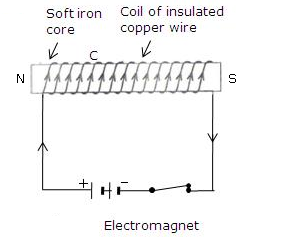A coil C of insulated copper wire is wound around a soft iron core NS and the two ends of the copper coil are connected to a battery. Thus, an electromagnet using a soft iron core.

(b) Electromagnetic cranes are used to separate copper from iron in a scrap yard. The current is switched on to energise the electromagnet and pick up the iron pieces from the scrap. Then these iron pieces are moved to another position, the electromagnet in switched off and the iron pieces are released.
Solution 21

(a) An electromagnet produces a magnetic field so long as current flows in its coil i.e., it produces temporary magnetic field.; but a permanent magnet produces a permanent magnetic field.

(b) Electromagnets: Electric bell, electric motors

Permanent magnets: Refrigerator doors, toys

Solution 22

(a) A solenoid is a long coil containing a large number of close turns of insulated copper wire.(b) The magnetic field produced by a current-carrying solenoid is similar to the magnetic field produced by a bar magnet.

(c) Magnetic field lines inside a current-carrying solenoid are in the form of parallel straight lines. This indicates that the magnetic field inside the solenoid is uniform.

(d) The magnetic field strength of a current-carrying solenoid can be increased by

(i) increasing the number of turns in the solenoid

(ii) increasing the current flowing through the solenoid

(iii) using soft iron as core in the solenoid

(e) Soft iron core

Solution 23

(a) An electromagnet is a temporary magnet that works on the magnetic effect of current. It consists of a long coil of insulated copper wire wrapped around a soft iron core that is magnetised ony when electric current is passed through the coil.

To make an electromagnet, we take a rod NS of soft iron and wind a coil C of insulated copper wire around it. When the two ends of the copper coil are connected to a battery, an electromagnet is formed.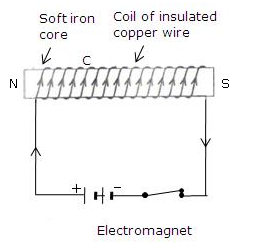(b) An electromagnet is called a temporary magnet because it produces magnetic field so long as current flows in its coil.

(c) Core of an electromagnet should be of soft iron and not of steel because soft iron loses all its magnetism when current in the coil is switched off but steel does not lose its magnetism when the current is stopped.

(d) Strength of electromagnet  depends on:

i. The number of turns in the coil - Increasing the number of turns in the coil increases the strength of the electromagnet.

ii. The current flowing in the coil - Increasing the current flowing in the coil increases the strength of the electromagnet.

iii. The length of air gap between its poles: Reducing the length of air gap between the poles of electromagnet increases the strength of the electromagnet.

(e) Electromagnets  are used in several electrical devices such as electric bell, electric motor, loudspeaker etc. They are also used by doctors to remove particles of iron or steel from a patient's eye and to remove pieces of iron from wounds.

Solution 5
Maxwell's right hand thumb rule.

## Chapter 2 - Magnetic Effects of Electric Current Exercise 84

Solution 37

(a) Clockwise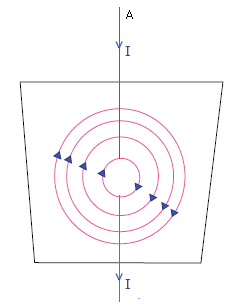(b) Anticlockwise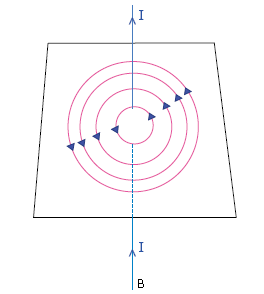We have used Maxwell's right hand thumb rule here.

Solution 38

End A will be a S-pole because current flows in the clockwise direction at A.

Solution 39

Direction of magnetic field is anticlockwise.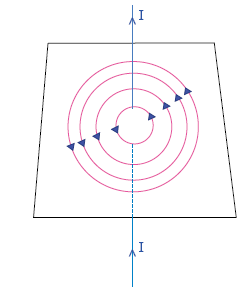Maxwell's right hand thumb rule. is used to find out the direction of magnetic field.

Solution 40

(a) End A becomes S-pole because current flows in clockwise direction at A.

(b) When A becomes S-pole, the other end becomes N-pole. So the tip of the compass (with also has North polarity) moves away from this end i.e., tip moves towards right.

Solution 41

Magnetic field lines around it will be clockwise (according to Maxwell's right hand thumb rule).

Solution 42

(a) End X is S-pole (because current flows in clockwise direction).

(b) End Y is N-pole (because current flows in anticlockwise direction).

(c) Clock face rule - Looking at the face of a loop, if the current around that face is in anticlockwise direction, the face has north polarity, while if the current at that face is in clockwise direction, the face has south polarity.

Solution 43

The direction of current will be from east to west.

We have applied MAxwell's right hand thumb rule here.

According to Maxwell's right hand thumb rule: Imagine that you are grasping the current-carrying wire in your right hand so that your thumb points in the direction of current, then the direction in which your fingers encircle the wire will give the direction of magnetic field lines around the wire.

Solution 44

(a) from top towards bottom

Solution 45

(a) Negative terminal

(b) Positive terminal

Because the current should be passed into wire upwards.

## Chapter 2 - Magnetic Effects of Electric Current Exercise 85

Solution 1
Weak ionic currents flowing in the human body produce magnetism.
Solution 2
The  technique called Magnetic Resonance Imaging (MRI) is based on magnetism produced in human body. It is used for obtaining images of internal parts of the body.
Solution 3
Brain and heart.
Solution 4
Magnetic Resonance Imaging
Solution 5
Magnetic Resonance Imaging (MRI)
Solution 6
Magnetic Resonance Imaging (MRI)

## Chapter 2 - Magnetic Effects of Electric Current Exercise 91

Solution 1

When a current-carrying conductor is placed in a magnetic field, a mechanical force is exerted on the conductor which can make the conductor move.

Solution 3
(a) Current

(b) Magnetic field

(c) Force acting on the conductor
Solution 4

Electrib bell works on the magnetic effect of current. It uses an electromagnet to produce sound.

Solution 5

Electrical motor

Solution 6

Electrical energy to mechanical energy.

Solution 7

False
An electric motor converts electrical energy into mechanical energy.

Solution 8
(a) Current - direction of center finger

(b) Magnetic field - direction of fore finger

(c) Force or Motion - direction of thumb
Solution 9
A commutator reverses the direction of current in the coil of a motor.
Solution 10

Commutator

Solution 11
The function of commutator rings is to reverse the direction of current flowing through the coil every time the coil just passes the vertical position during a revolution.
Solution 12

Carbon

Solution 13
The core of the coil of an electric motor made of soft iron.
Solution 15

The function of split rings is to reverse the direction of current flowing through the coil every time the coil just passes the vertical position during a revolution.

Solution 16

(a) left

(b) Commutator; rotation

Solution 17

(a) Fleming's left hand rule

(b) By increasing the current flowing in the conductor; by increasing the strength of magnetic field

(c) Electric motor

Solution 2
The force experienced by a current-carrying conductor placed in a magnetic field is the largest when the current carrying conductor is at right angles to the magnetic field.
Solution 14
Brush remains fixed. Commutator rotates with the coil.

## Chapter 2 - Magnetic Effects of Electric Current Exercise 92

Solution 18
Fleming's left hand rule: Hold the forefinger, the centre finger and the thumb of your left hand to right angles to one other. Adjust your hand in such a way that the forefinger points in the direction of magnetic field and the and the centre finger points in the direction of current, than the direction in which thumb points, gives the direction of force acting on the conductor.Solution 19
Principle of electric motor: When a rectangular current carrying coil is placed in a magnetic field, a force acts the coil which rotates it continuously. Electric motors are used in electric fans, washing machines, mixer, grinder etc.
Solution 20
(a) The current to the coil must be reversed twice during each rotation so that the coil keeps rotating continuously in the same direction.

(b) Commutator
Solution 21
(a)

(i) Direction of rotation would be reversed

(ii) Direction of rotation would be reversed

(iii) Direction of the rotation would remain unchanged

(b) Motor can be made more powerful by winding the coil on a soft iron core of by increasing the number of turns of the coil.
Solution 22

(a) Electric motor is a device used for converting electrical energy into mechanical energy.

Working of an electric motor: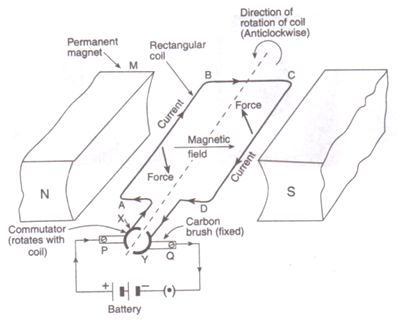Initially, the coil ABCD is in the horizontal position. On pressing the switch, current enters the coil through carbon brush P and commutator half ring X. The current flows in the direction ABCD and leaves via ring Y and brush Q. The direction of magnetic field is from N pole to S pole of the magnet. According to Fleming's left-hand rule, the force on sides AB and CD is in the downward and upward directions respectively. This makes the coil ABCD move in the anticlockwise direction.

When the coil reaches vertical position, then the brushes P and Q will touch the gap between the two commutator rings and current is cut off. But the coil does not stop rotating as it has already gained momentum. When the coil goes beyond the vertical position, the side CD comes on the left side and side AB comes to the right side, and the two commutator rings change contact from one brush to the other. This reverses the direction of current in the coil, which in turn reverses the direction of forces acting on the sides AB and CD of the coil. The side CD is pushed down and side AB is pushed up. Thus, the coil rotates anticlockwise by another half rotation.

The reversing of current in the coil is repeated after every half rotation due to which the coil (and its shaft) continues to rotate as long as current from the battery is passed through it. The rotating shaft of electric motor can drive a large number of machines which are connected to it.

(b) In commercial electric motors:

i. the coil is wound on a soft iron core. This increases the strength of magnetic field, which makes the motor more powerful.

ii. the coil contains a large number of turns of insulated copper wire.

iii. a powerful electromagnet is used in place of permanent magnet.

## Chapter 2 - Magnetic Effects of Electric Current Exercise 93

Solution 31

Clockwise direction (according to Fleming's left hand rule).

Solution 32

According to Fleming's left hand rule, the wire moves in the upward direction (out of the page).

Solution 33
Force will be due South (according to Fleming's left hand rule).
Solution 34
According to Fleming's left hand rule, the wire moves in the downward direction (into the page).
Solution 36

Positive charge

Solution 35

Force on a current-carrying wire that is parallel to magnetic field will be zero.

This is because the magnitude of force depends on the sin of the angle between the direction of current and the direction of magnetic field, so if the current carrying wire is held parallel to the magnetic field, the force will be zero.

## Chapter 2 - Magnetic Effects of Electric Current Exercise 102

Solution 1

Electric Generator

Solution 2

(a) D.C. Generator

(b) A.C. Generator

Solution 3

Electromagnetic Induction

Solution 4
Fleming's Right Hand Rule
Solution 5
The condition necessary for the production of current by electromagnetic induction is that there must be a relative motion between the wire and the magnet.

## Chapter 2 - Magnetic Effects of Electric Current Exercise 103

Solution 6

AC Generator (or Alternator)

Solution 7

If we replace the slip rings of an AC generator by a commutator, then it will become a DC generator.

Solution 8

(a) True

(b) False

Solution 9

Function of brushes is to transfer the current from coil to load.

Solution 10

This phenomena is known as electromagnetic induction.

Solution 11

Electromagnetic induction

Solution 12

Simple alternator:- Magnet fixed and coil rotates;

Practical alternator:- Coil fixed and magnet rotates.

Solution 13
To obtain water for making steam for turning turbines and for cooling spent steam to condense it back into hot water for making fresh steam.
Solution 14

Electromagnet, permanent magnet, wire carrying current.

Solution 15

direct

Solution 16
Yes, some current will be induced in the coil B because of change in magnetic field through the coil B due to change in current in coil A. This is called electromagnetic induction.
Solution 17

(a) Electric generator is based on the principle that when a straight conductor is moved in a magnetic field, then current is induced in the conductor.

(b) Two ways in which the current induced in the coil of a generator could be increased are:

(i) by roating the coil faster

(ii) by using a coil with a larger area

Solution 18
(a) The difference between AC and DC is that DC flows in one direction only while AC reverses direction after equal intervals of time.

(i) DC current remains same with time in its value and direction.

(ii) AC current changes with time and changes its direction every time after a certain interval of time.

(b)

(i) DC

(ii) AC

Solution 19

The direction of induced current produced in a straight conductor moving in a magnetic field is given by Fleming's right hand rule.

According to Fleming's right hand rule : Hold the thumb, the fore finger and the centre finger of your right-hand at right angles to one another. Adjust your hand in such a way that forefinger points in the direction of magnetic field, and thumb points in the direction of motion of conductor, then the direction in which centre finger points, gives the direction of induced current in the conductor.Solution 20

(a) Fleming's right hand rule:- Hold the thumb, the fore finger and the centre finger of your right-hand at right angles to one another. Adjust your hand in such a way that forefinger points in the direction of magnetic field, and thumb points in the direction of motion of conductor, then the direction in which centre finger points, gives the direction of induced current in the conductor.(b) Fleming's left hand rule:- Hold the forefinger, the centre finger and the thumb of your left hand at right angle to one another. Adjust your hand in such a way that the forefinger points in the direction of magnetic field and the and centre finger points in the direction of current, then the direction in which thumb points, gives the direction of force acting on the conductor.Solution 21
(a). Construction-wise, the only difference between a D.C. generator and an A.C. generator is in the way the two ends of the generator coil are linked to the outer circuit. In a D.C. generator we connect the two ends of the coil to a commutator consisting of two half rings of copper. In an A.C. generator we connect the two ends of the coil to two full rings of copper called slip rings. There is no commutator in an A.C. generator.

(b) Generally, the alternators in a Thermal Power Station are driven by the power of high pressure steam.

To heat water in the boiler, fuels like coal or natural gas can be used.
Solution 22Construction:

A simple AC generator consists of a rectangular coil ABCD which can be rotated rapidly between the poles N and S of a strong horseshoe-type permanent magnet M. The coil is made of a large number of turns of insulated copper wire. The two ends A and D of the coil are connected to two circular pieces of copper metal called slip rings  R1 and R2. As the slip rings rotate with the coil, the two fixed pieces of carbon called brushes, B1 and B2, keep contact with them. So, the current produced in the rotating coil can be tapped out through slip rings into the carbon brushes. The outer ends of carbon brushes are connected to a galvanometer to show the flow of current in the external circuit.

Working:

Suppose the coil ABCD, which is initially in the horizontal position, is rotated in the anticlockwise direction. The side AB of the coil moves down and side CD moves up. Due to this, induced current is produced in both the sides, which flows in the direction BADC (according to Fleming's right hand rule). Thus, in the first half rotation, the current in the external circuit flows from brush B1 to B2. After half revolution, sides AB and CD will interchange their positions. So, side AB starts moving up and side CD starts moving down. As a result, direction of induced current in the coil is reversed and flows in the direction CDAB. The current in the external circuit flows from brush B2 to B1.

Solution 23

(a) The production of electricity from magnetism is known as electromagnetic induction.

Let us move a wire AB upward rapidly between the poles of the horseshoe magnet. When the wire is moved up, there is a deflection in the galvanometer pointer which shows a current is produced in the wire AB momentarily. Thus, as the wire is moved up through the magnetic field, an electric current is produced in it.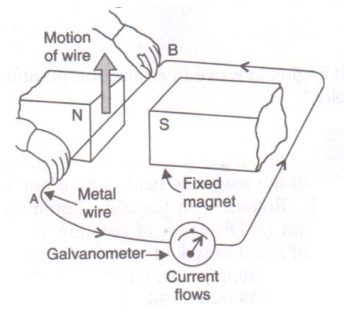(b) Electric generator

(c) Different ways to induce current in a coil of wire are:

(i) by moving the coil relative to a fixed magnet

(ii) by keeping the coil fixed and moving a magnet relative to it.

Solution 24
(a) If the current flows in one direction only, it is known as direct current; and if the current reverses direction after equal intervals of time, it is called alternating current.

(b) Source of DC are dry cell, car battery, DC generator etc.

Source of AC are AC generator, bicycle dynamos etc.

(c) An important advantage  of AC over DC is that AC can be transmitted over long distances without much loss of electrical energy.

(d) 50Hz

## Chapter 2 - Magnetic Effects of Electric Current Exercise 105

Solution 37

(a) The galvanometer deflects to the left.

(b) The galvanometer deflects to the left.

(c) No deflection in galvanometer.

The deflection in the galvanometer can be increased by

i. increasing the number of turns in the coil

i. using a strong magnet

ii. increasing the speed with which magnet is moved in the coil.

Solution 38

(i) Electromagnetic induction.

(ii) The galvanometer gives a reading to the left.

(iii) Large deflection to right occurs more quickly.

Solution 39
Relative motion between the coil and the magnet.
Solution 40

(a) Current increased

(b) Current reversed

(c) Current increased

(d) Zero current

(e) Zero current

Solution 41

(i) Galvanometer pointer moves to one side showing that a momentary current is induced in the coil A.

(ii) Galvanometer pointer moves to the other side showing that the direction of momentarily induced current has been reversed.

The phenomenon taking place here is electromagnetic induction. When the current is passed through coil B or is stopped, the magnetic field linked with coil A changes due to which an induced current is produced in the coil.

Solution 42
Step-down transformer (which reduces the voltage).

## Chapter 2 - Magnetic Effects of Electric Current Exercise 113

Solution 5

Earth wire

Solution 1
Electric fuse
Solution 2

(i) 5A

(ii) 15A
Solution 3Solution 4
(a) False

(b) False

## Chapter 2 - Magnetic Effects of Electric Current Exercise 114

Solution 6

Red wire - Live wire

Black wire - Neutral wire

Green wire - Earth wire

Solution 7

Zero volt

Solution 8

P = VI

I = P/V = 180/240 = 0.75A

The fuse wire should be such that it is able to withstand only a little more current than 0.75A. So the fuse of 1A is the most suitable.

Solution 9
Short circuit occurs when live wire and neutral wire come in contact with each other.
Solution 10

Live wire

Solution 11

In series.

Solution 12
Red, black and green.
Solution 13

(a)red

(b)black

(c)green

Solution 14

To avoid the risk of electric shocks.

Solution 15
(i) In case of parallel connection, if one of the appliances is switched off, other appliances keep on operating.

(ii) In case of parallel connection, all the appliances are operated on same voltage i.e., the mains supply voltage.
Solution 16

Parallel connection

Solution 17

(a) live

(b) body

Solution 18
(a) The fuse wire is made of tin-plated copper wire because of its low melting point.

(b) Pure copper wire cannot be used as a fuse wire because  it has a high melting point due to which it will not melt easily when a short circuit takes place.
Solution 19

Cartridge fuse.

It consists of a glass tube T having a thin fuse wire sealed inside it. The glass tube has two metal caps at its two ends. The two ends of the fuse wire are connected to these metal caps. The metal caps are for connecting the fuse in the circuit in a suitably made bracket.Solution 20

Live wire coming in contact with the neutral wire is known as short circuit.

When too many electrical appliances of high power rating are switched on at the same time or are connected to a single socket, they draw extremely large current. This is known as overloading.

Solution 21

(a) A fuse cuts off current when the current exceeds a safe value (due to short circuiting or overloading). When the current becomes large, it heats the fuse wire too much. Since the melting point of fuse wire is low, it melts and breaks the circuit. Thus, current in the circuit is cut off.

(b) Let the maximum number of bulbs be y.

Power of y bulbs, P=60y

V=220V, I=5A

We know that

P = VI

60y = 220 x 5

60y = 1100

y=18.33

So, number of bulbs required are 18.

Solution 22
(i) A fuse is one of most important protection devices, which is used for avoiding the damages happening due to over load or short circuit.

(ii) An earthing wire is used to save us from the risk of electric shock in case the live wire touches the metal case of the electric appliance.
Solution 23

(a) V=230V, P=750 W, t=30/60=0.5hr

(i) Let max current be I

We have P=VI

750 =230xI

I= 3.26 A

(ii) Electric energy consumed, E = Pxt = 0.75kW x 0.5h = 0.375 kWh

No. of units used in 30 min = 0.375

(b) 5 A fuse rating will be suitable for this electric iron as the maximum current for this iron is 3.26 A.

Solution 24

When the live wire of a faulty appliance comes in direct contact with its metallic case, which has been earthed, the large current passes directly to the earth without passing through the user's body. Thus, it is necessary to earth the metallic bodies of electrical appliances so as to avoid fatal electric shocks.

Solution 25

(a) P = 3kW = 3000W

V = 240V

P = V x I

I = P/V

= 3000/240 = 12.5 A

(b) A 13A fuse should be used in the geyser circuit.

Solution 26
(a) Fuses are fitted in the fuse box of a domestic electricity supply to protect the whole wiring of the house when excessive current flows in the circuit.

(b) MCB
Solution 27

(a)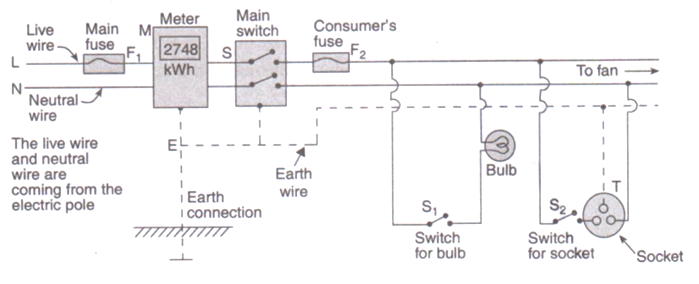(b) Two hazards associated with the use of electricity are:

i. If a person happens to touch a live electric wire, he gets a severe electric shock.

ii. Short-circuiting due to damanged wiring or overloading of the circuit can cause electrical fire in a building.

(c) Important precautions which should be observed in the use of electricity are:

(i) Use of good quality wires

(ii) Use of fuse and proper earthing.

(iii) Use of appliances in dry condition only.

(d) If a person comes in contact with a live wire, we will switch off the main switch immediately so as to cut off the electricity supply..

(e) Electric switches should not be operated with wet hands because water is a good conductor of electricity, so the user may get electric shock.

## Chapter 2 - Magnetic Effects of Electric Current Exercise 115

Solution 38

Given: P=3.2kW=3200W, Fuse current rating=10 A, V=220 V

We have

P=VI

3200 = 220 x I

I=14.54 A

As the required current for the air-conditioner is 14.54A and the rated current of the fuse is 10A, so the fuse will blow cutting off the power supply.

Solution 39

P1=60 W, P2=1200W, P3=500W

Fuse rating = 10A

V=220V

We have, P=VI

Total power=60+1200+500=1760W

Therefore, 1760=220xI

I=8A

The required current is 8A and fuse rating is 10A. So, all the appliances will work normally and the fuse will not blow.

Solution 40
(a) The appropriate value of the fuse to be fitted in the circuit is 2.5A or 3A.

(b) If a 13A fuse is fitted in the circuit, it will not protect the vacuum cleaner against the very high current flowing through it. This may damage the appliance.
Solution 41

Circuit A is not dangerous after fuse blows because fuse is fitted in the live wire; but circuit B is dangerous even if fuse blows because the fuse is in the neutral wire.

## Chapter 2 - Magnetic Effects of Electric Current Exercise 116

Solution 42

P1=1200W, P2=1000W

V=220V

Fuse rating=9A

We know, P=VI

Total current required,

I=P/V

=(P1+P2)/V

=(1200+1000)/220

=10A

If both the appliances are switched on together, the fuse will get burnt. So, both the appliance cannot be used at same time.

Solution 43

No earth connection is required for the bulb connection as it does not draw heavy current and we hardly touch it. A socket for using an electric iron has an earth connection because electric iron has a metallic body and draws a large current.

Solution 44
(a) It is more dangerous to touch the live wire rather than the neutral wire because live wire has a high potential of 220V, where as neutral wire has zero potential.
(b) Bird's body is not connected to the earth, so no current flows through its body into the earth. So, it is safe for birds to sit on naked power lines fixed atop tall electric poles.
Solution 45
Let the maximum number of tube-lights be y.

Power of y tube-lights, P=36y

V=230V, I=5A

We know that

P = VI

36y = 230 x 5

36y = 1500

y=31.94

So, number of tube-lights required are 31.

### STUDY RESOURCES

REGISTERED OFFICE : First Floor, Empire Complex, 414 Senapati Bapat Marg, Lower Parel, Mumbai - 400013, Maharashtra India.